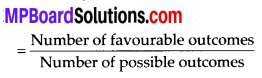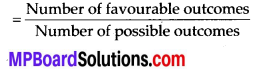# MP Board Class 7th Maths Solutions Chapter 3 Data Handling Ex 3.4

## MP Board Class 7th Maths Solutions Chapter 3 Data Handling Ex 3.4

Question 1.
Tell whether the following is certain to happen, impossible, can happen but not certain.
(i) You are older today than yesterday.
(ii) A tossed coin will land heads up.
(iii) A die when tossed shall land up with 8 on top.
(iv) The next traffic light seen will be green.
(v) Tomorrow will be a cloudy day.
Solution:
(i) Certain to happen
(ii) Can happen but not certain
(iii) Impossible as there are only six faces of a die marked as 1, 2, 3, 4, 5 and 6 on it.
(iv) Can happen but not certain
(v) Can happen but not certainQuestion 2.
There are 6 marbles in a box with numbers from 1 to 6 marked on each of them.
(i) What is the probability of drawing a marble with number 21
(ii) What is the probability of drawing a marble with number 5?
Solution:
Probability of an event(ii) Probability of drawing a marble with number 5 = $$\frac{1}{6}$$.

Question 3.
A coin is flipped to decide which team starts the game. What is the probability that your team will start?
Solution:
A coin has two faces – Head and Tail. One team can opt either Head or Tail. Probability of an eventProbability (our team will start first) = $$\frac{1}{2}$$